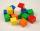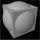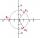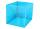# Pythagorean theorem + cube - math problems

#### Number of problems found: 39

• Body diagonalThe cuboid has a volume of 32 cm3. Its side surface area is double as one of the square bases. What is the length of the body diagonal?
• Cube and sphereCube with the surface area 150 cm2 is described sphere. What is sphere surface?
• Chocolate rollThe cube of 5 cm chocolate roll weighs 30 g. How many calories will contain the same chocolate roller of a prism shape with a length of 0.5 m whose cross-section is an isosceles trapezoid with bases 25 and 13 cm and legs 10 cm? You know that 100 g of this
• Wall diagonalCalculate the length of wall diagonal of the cube whose surface is 384 cm square.
• Pyramid in cubeIn a cube with an edge 12 dm long, we have an inscribed pyramid with the apex at the center of the cube's upper wall. Calculate the volume and surface area of the pyramid.
• Sphere vs cubeHow many % of the surface of a sphere of radius 12 cm is the surface of a cube inscribed in this sphere?
• Cube diagonalsDetermine the volume and surface area of the cube if you know the length of the body diagonal u = 216 cm.
• CubeCalculate the surface of the cube ABCDA'B'C'D' if the area of rectangle ACC'A' = 344 mm2.
• Body diagonalFind the cube surface if its body diagonal has a size of 6 cm.
• Cube - wallV kocke ABCDEFGH je |CF|=4 √ 2. Aký je povrch kocky?
• Cube from sphereWhat largest surface area (in cm2) can have a cube that we cut out of a sphere with radius 43 cm?
• Moivre 2Find the cube roots of 125(cos 288° + i sin 288°).
• Center of the cubeThe Center of the cube has a distance 16 cm from each vertex. Calculate the volume V and surface area S of the cube.I have homework. The cube has an edge 7 cm long and I must find wall and body diagonal.
• PrismRight-angled prism, whose base is a right triangle with leg a = 3 cm and hypotenuse c = 13 cm, has the same volume as a cube with an edge length of 3 dm. a) Find the height of the prism b) Calculate the surface of the prism c) What percentage of the cube'
• Cube in a sphereThe cube is inscribed in a sphere with a volume 7253 cm3. Determine the length of the edges of a cube.
• CubesOne cube is an inscribed sphere and the other one described. Calculate the difference of volumes of cubes, if the difference of surfaces in 257 mm2.
• Cube - anglesCalculate the angle between the wall diagonal and cube base. Calculate the angle between the cube body diagonal and cube base.
• Cube diagonalDetermine the length of the cube diagonal with edge 37 mm.

Do you have an exciting math question or word problem that you can't solve? Ask a question or post a math problem, and we can try to solve it.

We will send a solution to your e-mail address. Solved examples are also published here. Please enter the e-mail correctly and check whether you don't have a full mailbox.

Pythagorean theorem is the base for the right triangle calculator. Pythagorean theorem - math problems. Cube Problems.